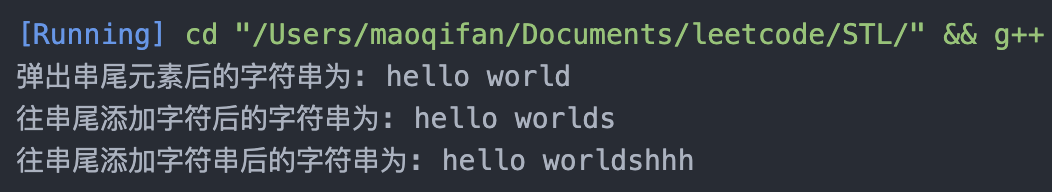# C++中 string 中的常用方法使用心得

更新时间：2020年03月26日 08:58:48   作者：maoqifan

string 字符串在所有的语言中都非常重要，c++也不例外,接下来我们将介绍string中的常用方法

1. size() 和 length() 函数 : 他们返回字符串的真实长度，且不会因为空格而截断，这两个方法完全等价，使用及输出如下：

```#include<iostream>
#include<string>
using namespace std;

int main(void)
{
string s = "dasddasd";
printf("size()返回的长度为:%lu\nlength()返回的长度为:%lu",s.size(),s.length());
return 0;
}```2. find()函数和rfind()函数 : 这两个函数用于查找字串在母串中的位置，并且返回该位置，当然如果找不到就会返回一个特别的标记`string::nops`,而find()函数是从字符串开始指针向后进行查找,rfind()函数是从字符串的结束指针开始向前查找，其使用及输出如下：

```#include<iostream>
#include<string>
using namespace std;

int main(void)
{
string s = "hello worldh";
int index = s.find("h");   // 从串首向后查找
int index2 = s.find("h",2)  // 固定位置后子串在母串的位置
int index1 = s.rfind("h"); // 从串尾向前查找
printf("(find()):字母h在母串中的位置为:%d\n", index);
printf("(rfind()):字母h在母串中的位置为:%d", index1);
return 0;
}``````#include<iostream>
#include<string>
using namespace std;

inline bool whetherOnly(string &str,string &base){
return base.find(str) == base.rfind(str);
}```

3. `find_last_of()`函数和`find_first_of()`函数：从函数名我们也可以知道`find_last_of()`函数是找这个子串在母串中最后一次出现的位置并且将该位置返回；而`find_first_of()`函数是找这个子串在母串中最后一次出现的位置并将该位置返回，其使用及输出如下:

```#include <iostream>
#include <string>
using namespace std;

int main(void)
{
string s = "hello worldh";

int index = s.find_first_of("h");
int index1 = s.find_last_of("h");
printf("(find_first_of()):字母h在母串中的位置为:%d\n", index);
printf("(find_last_of()):字母h在母串中的位置为:%d", index1);
}```4.assign()函数：该函数用于将目标串的值复制到该串上，并且只复制值，其使用及输出如下：

```#include <iostream>
#include <string>
using namespace std;

int main(void)
{
string s = "hello worldh";
s.clear();
s.assign("hello world");
cout<<s<<endl;

}```5.clear()函数，把当前字符串清空，这时候如果调用`string::size()`函数或`string::length()`函数将返回0，其使用及输出如下：

```#include <iostream>
#include <string>
using namespace std;

int main(void)
{
string s = "hello worldh";
s.clear();
cout<<"clear后的串的长度"<<s.size()<<endl;
}```6.resize()函数，该函数可以将字符串变长到指定长度，若小于原本字符串的长度，则会截断原字符串；这个函数的一个重载形式是`str.resize(length,'s')` 可以用该输入字符's'来对字符串进行扩充至length的长度，该函数的使用及输出如下：

```#include <iostream>
#include <string>
using namespace std;

int main(void)
{
string s = "hello worldh";
s.resize(5);    // s会变为 hello
cout<<s<<endl;
s.resize(10,'C'); // s 会变为 helloCCCCC
cout<<s<<endl;

}```7. `replace(pos,len,dist)`函数: 该函数用于将该串从pos位置开始将长度为len的字串替换为dist串,值得注意的是该函数只替换一次，这与市面上的py和java等语言不一样，需要留意，该函数的使用和输出如下：

```#include <iostream>
#include <string>
using namespace std;

int main(void)
{
string s = "hello worldh";
s.replace(s.find("h"),2,"#"); // 把从第一个h开始的两个字符变为一个字符 #
cout<<"替换后的字符串为: "<<s<<endl;

}``````// 替换字符串里的所有指定字符
string replace(string &base, string src, string dst) //base为原字符串，src为被替换的子串，dst为新的子串
{
int pos = 0, srclen = src.size(), dstlen = dst.size();
while ((pos = base.find(src, pos)) != string::npos)
{
base.replace(pos, srclen, dst);
pos += dstlen;
}
return base;
}```

8. `erase(index,length)`函数:该函数删除index位置后length长度的子串，其代码及输出如下:

```#include <iostream>
#include <string>
using namespace std;

int main(void)
{
string s = "hello worldh";
s.erase(s.find("h"),3);
cout<<"擦除过后的串"<<s<<endl; // 将会输出lo worldh
}```9.substr(index,length)函数:该函数从index开始截断到长度为length并返回截断的子串；值得注意的是，该函数不改变母串的值，其使用及输出如下:

```#include <iostream>
#include <string>
using namespace std;

int main(void)
{
s = s.substr(0,5);
cout<<"截断并赋值后的字符串为:"<<s<<endl; // 会输出hello
}```10 . push_back(char c)函数，pop_back()函数，append(string s)函数：push_back(char c)函数往该字符串的尾端加入一个字符;pop_back()函数从该字符串的尾端弹出一个字符;而apend(string s)函数将会在该字符串的末尾添加一个字符串，并且返回添加后字符串的引用。他们的使用及输出如下图所示:

```#include <iostream>
#include <string>
using namespace std;

int main(void)
{
string s = "hello worldh";
// s.erase(s.find("h"),3);
s.pop_back(); //弹出串的最后一个元素
cout<<"弹出串尾元素后的字符串为: "<<s<<endl;
s.push_back('s'); // 在串的最后添加一个字符
cout<<"往串尾添加字符后的字符串为: "<<s<<endl;
s.append("hhh"); // 在串的最后添加一个字符串
cout<<"往串尾添加字符串后的字符串为: "<<s<<endl;
}``````// 给你一份『词汇表』（字符串数组） words 和一张『字母表』（字符串） chars。
// 假如你可以用 chars 中的『字母』（字符）拼写出 words 中的某个『单词』（字符串），那么我们就认为你掌握了这个单词。

// 注意：每次拼写时，chars 中的每个字母都只能用一次。
// 返回词汇表 words 中你掌握的所有单词的 长度之和。

// 输入：words = ["cat","bt","hat","tree"], chars = "atach"
// 输出：6
// 解释：
// 可以形成字符串 "cat" 和 "hat"，所以答案是 3 + 3 = 6。

// 输入：words = ["hello","world","leetcode"], chars = "welldonehoneyr"
// 输出：10
// 解释：
// 可以形成字符串 "hello" 和 "world"，所以答案是 5 + 5 = 10。

#include <iostream>
#include <vector>
#include <string>
using namespace std;
class Solution
{
public:
int countCharacters(vector<string> &words, string chars)
{
int count = 0;
bool flag = false;           // 标记
string c_chars(chars);         // 构造c_chars保存chars
for (int i = 0; i < words.size(); i++) // 迭代单词表
{
if (c_chars.size() < words[i].size()) //如果单词的字母多于可选字母，则跳过这个单词
continue;
for (int j = 0; j < words[i].size(); j++) // 迭代可选择的字母
{
int index = c_chars.find(words[i][j]);
if (index != c_chars.npos) // 能找到这个字母
{
flag = true;
c_chars.erase(index, 1); // 从c_chars()删除这个字母
}
else
{
flag = false; // 不能找到,意味着不能组成这个单词
break;    //跳出这次循环
}
}
if (flag) // 如果符合则计数加1
count += words[i].size();
c_chars.assign(chars); // 把chars的值在再次赋值给c_chars
}
return count;
}
};```